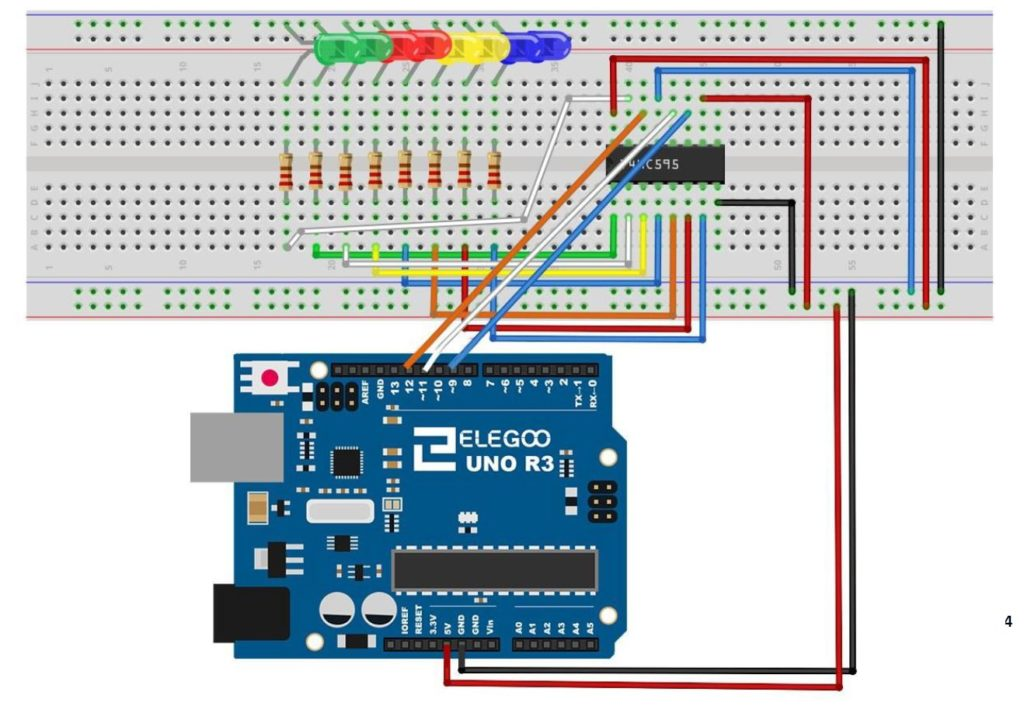# Arduino Tutorial 45: Understanding Circular Shift Left and Circular Shift Right with the 74HC595

In this lesson we explore the Circular Shift Left (CSL) and Circular Shift Right (CSR)  binary functions. These functions are similar to the Logical Shift Left and Logical Shift Right functions explained in the previous lesson.

As a reminder, we are doing this work on the circuit built in Tutorial 42. As a refresher, we are using the 74HC595 Serial to Parallel converter, connected to an Arduino according to the following schematic.This is the schematic we use in this example to control 8 LEDs from the 74HC595 chip.

In all these lessons we are using the Arduino Super Starter Kit, which you can pick up HERE.

The Circular Shift Left function is illustrated below:

You can see that all bits shift to the left, with the Most Significant Bit, or MSB looping back to the Least Significant Bit, or LSB position.

Similarly, the Circular Shift Right Function is as follows:

There is actually a very easy way to implement this in a program. We illustrate it with an arduino program. Lets first consider the CSL function. We will first consider how to move the MSB to the LSB position. Remember from our earlier lessons, that dividing a binary number by 2 moves the bits to the right. We want to move the MSB 7 positions to the right, so we would divide by 27, which is 128.  So, if we have a binary number myByte, we could move the MSB to the LSB by the equation:

myByte=myByte/128

With this we do the hardest part, which is to get the MSB to the LSB. Now we need to get the rest of the bits back, and in the left shifted position, we do that by multiplying by two. By combining these two functions, we end up with the solution of CSL being:

myByte=myByte/128+myByte*2

This simple equation will perform the CSL operation, when myByte is a Hexadecimal or Binary number (Byte type in Arduino).

We show the code below to implement the CSL an arduino with a 74HC595 chip:

Similarly, the CSR binary function can be achieved with the equation:

myByte=myByte*128+myByte/2

This is the code we use for CSR on the Arduino.

# Arduino Tutorial 44: Understanding Logical Shift Left and Logical Shift Right with the74HC595

In this lesson, we explore how to perform Logical Shift Left (LSL), and Logical Shift Right (LSR) functions on binary numbers, and we implement a circuit to perform these functions using an Arduino and a 74HC595 chip. We will demonstrate these functions on 8 bit binary numbers.

We start with the basic circuit and code which were developed in Lesson 42. In this lesson we are using parts from the Elegoo Arduino kit, which you can get  HERE.  We start with this circuit, which was explained in Lesson 42.This is the schematic we use in this example to control 8 LEDs from the 74HC595 chip.

You can see that with this circuit, an 8 bit binary number can be visually displayed by illuminating the circuit LED. The goal of this lesson is to write code to perform LSL and LSR functions. The graphics below show conceptually how simple these functions are:

MSB stands for “Most Significant Bit” and LSB stands for “Least Significant Bit”.  You can see that the LSL function just moves each bit one to the left, and fills the empty LSB with a “0”.

The LSR funtion is just as simple as illustrated below.

Such shifts are often required when doing digital logic, so it is important to understand what the terms mean.

We can see that the LSL function can be performed by simply multiplying the binary number by 2. Similarly the LSR function can be performed by dividing the binary number by 2.

Code for LSL:

Code for LSR function:

# Arduino Tutorial 43: Binary Counter with 74HC595 Serial to Parallel Shift Register

In lesson 42 we showed you how to connect and program the 74HC595 shift register. We showed how data in byte format would then be written to an array of 8 LED to give a visual representation of the binary version of that byte variable. We then gave you the assignment to create a Binary Counter using the 4HC595. In this lesson we show you the solution. This builds on Lesson 42, so make sure to have your basic 74HC595 circuit set up before starting this lesson.

In this lesson we are using parts from the Elegoo Arduino kit, which you can get  HERE.

The code we ended up developing in this lesson is provided below.